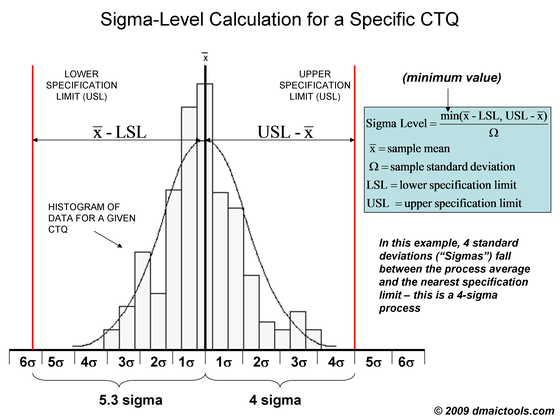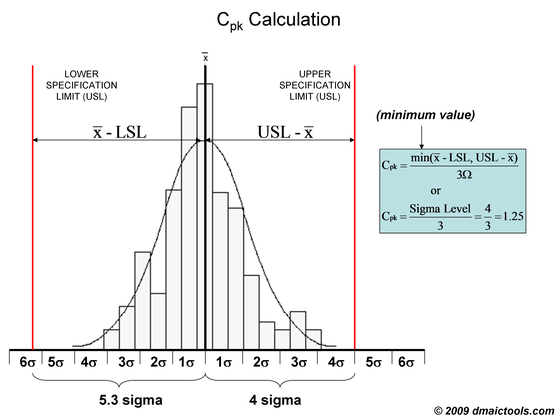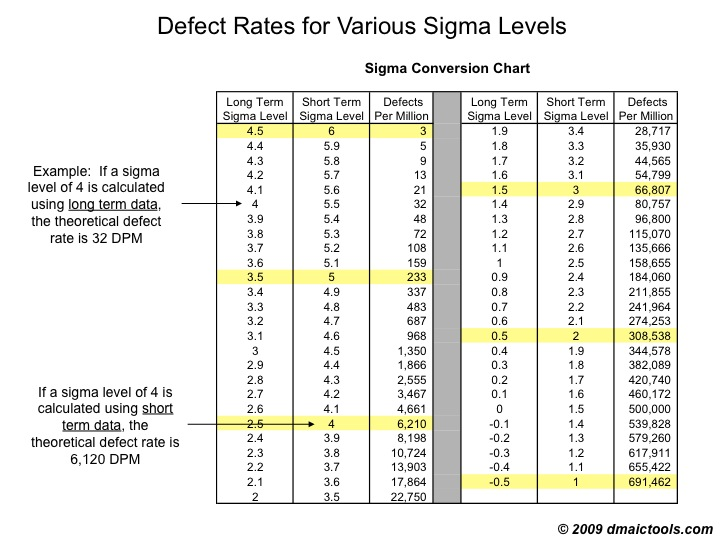# Sigma Level

Process sigma (also referred to as sigma level) is a measure of process capability:  the higher the process sigma, the more capable the process is.  A Six Sigma process has a short-term process sigma of 6, and a long-term process sigma of 4.5 (see why not 4.5 sigma?).  The theoretical defect rate for a Six Sigma process is 3.4 defects per million (DPM).

Simply put, the process sigma indicates how many standard deviations (“Sigmas”) can fit inside the gap between the process average and the nearest specification limit:Note that the above example shows a histogram for a particular CTQ, so the process sigma of 4.5 applies to the specific CTQ being studied.

If an overall long-term defect rate is available for all defects, it is possible to state the process sigma for the entire process (all CTQ’s and their associated defects) by locating the defect rate on the Sigma Conversion Chart and finding the corresponding sigma-level.  Typically, however, a Six Sigma project will be sufficiently narrowed to focus on one or two CTQ’s , which will be evaluated separately for their process sigma levels.

If an overall long-term defect rate is available for all defects, it is possible to state the process sigma for the entire process (all CTQ’s and their associated defects) by locating the defect rate on the Sigma Conversion Chart and finding the corresponding sigma-level.  Typically, however, a Six Sigma project will be sufficiently narrowed to focus on one or two CTQ’s , which will be evaluated separately for their process sigma levels.

In cases where both lower and upper specification limits exist and the process is not capable on either side of the distribution, the process sigma can be calculated by adding the theoretical DPM levels on each side of the distribution (using the Sigma Conversion Chart) and then finding the corresponding process sigma for the combined DPM level.

A more common measure of process capability is Cpk, which is equal to the process sigma divided by 3.  So a Six Sigma process has a Cpk of 2.0.What are the defect levels associated with various process sigma levels?

A Sigma Conversion Chart provides the theoretical defect rates associated with various sigma-levels.  The conversion chart assumes that the underlying data is continuous and normally distributed, as in the histogram above.## Long-Term Versus Short-Term Data

Notice that the above chart includes columns for long-term and short-term process sigma levels, depending on how extensive our sample data set is.  Use the short-term column if data has been collected over a limited period of time, and the long-term column if data is extensive and likely includes all variation sources that can influence the CTQ over the long run.

Show less...